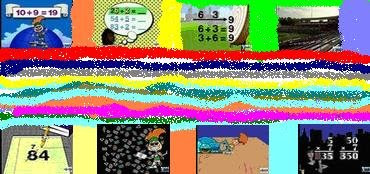## sábado, septiembre 20, 2008

### Recurso: Autismo y matemáticas II

.
.SUBTRACTION

MULTIPLICATION

DIVISION

# Web Resources

WebMath
Explores topics in arithmetic, K-8 math, trigonometry, calculus, and everything in between. Features a drop-down menu with links to the most common math questions.

Math.com Homework Help
Offers help on topics in prealgebra, algebra, and geometry.

AAA Math
Provides a comprehensive set of interactive math lessons for grades 1-8. Subjects include addition, subtraction, counting, estimation, exponents, fractions, graphs, mental math, geometry, and statistics.

Harcourt Animated Math Glossary
Defines important math terms for grades 1-6, from congruent figures to ordinal numbers.

Math Dictionary for Kids
Includes definitions of concepts from arithmetic, algebra, and geometry.